Home > jstse/mvpp exams > JSTSE | MVPP 2020-21 Question Paper With Answer Keys and Solutions

# JSTSE | MVPP 2020-21 Question Paper With Answer Keys and Solutions# JSTSE | MVPP 2020-21 Question Paper With Answer Keys And Solutions

Hi students, welcome to AmansMathsBlogs (AMB). On this article, you will get JSTSE MVPP 2020-21 Question Paper With Answer Keys And Solutions.

JSTSE 2020-21 is an exam that is conducted by science branch of the Directorate of Education, Government of Delhi. This exam is scheduled in Dec or Jan month of the session in every year. But, due to Covid-19 in India, this year, in 2020-21 session, JSTSE is conducted on 28 March 2021 (Sunday). From 2021, JSTSE (Junior Science Talent Search Examination) is now known as MVPP (Mukhyamantri Vigyan Pratibha Pariksha).

JSTSE / MVPP Previous Year Questions Papers with Solutions
JSTSE 2020-21 Question Papers with Answer Keys & Solutions
JSTSE 2019-20 Question Papers with Answer Keys & Solutions
JSTSE 2018-19 Question Papers with Answer Keys & Solutions
JSTSE 2017-18 Question Paper Not Available
JSTSE 2016-17 Question Papers with Answer Keys & Solutions
JSTSE 2015-16 Question Papers with Answer Keys & Solutions
JSTSE 2014-15 Question Papers with Answer Keys & Solutions
JSTSE 2013-14 Question Papers with Answer Keys & Solutions
JSTSE 2012-13 Question Papers with Answer Keys & Solutions
JSTSE 2011-12 Question Papers with Answer Keys & Solutions
JSTSE 2010-11 Question Papers with Answer Keys & Solutions
JSTSE 2009-10 Question Papers with Answer Keys & Solutions
JSTSE 2008-09 Question Papers with Answer Keys & Solutions
JSTSE 2007-08 Question Papers with Answer Keys & Solutions
JSTSE 2006-07 Question Papers with Answer Keys & Solutions
JSTSE Physics Questions with Answer Keys & Solutions
JSTSE Chemistry Question with Answer Keys & Solutions
JSTSE Biology Question with Answer Keys & Solutions
JSTSE Maths Question with Answer Keys & Solutions

The math questions of JSTSE MVPP 2020-21 Question Paper With Answer Keys And Solutions are solved by me, AMAN RAJ, I am maths faculty of IIT Maths Foundation course and founder of this website AmansMathsBlogs (AMB).

Read More : 129 Maths Short Tricks

## JSTSE MAT 2020-21 Answer Keys: PART – I

JSTSE | MVPP MAT Answer Keys
Ques NoAns KeyQues NoAns KeyQues NoAns KeyQues NoAns Key
14262513762
22273522771
31284534781
44293541793
52301552804
61313562812
73323573822
84331583833
93342592843
103353603853
114364614864
123371623872
134382632884
143392642891
153403653904
162411664912
171421671923
183433684934
192443692942
204452704952
212462711964
224474722972
234482731983
242494741991
2515027531002

## JSTSE SAT 2020-21 Answer Keys: PART – II

JSTSE | MVPP SAT Answer Keys
Ques NoAns KeyQues NoAns KeyQues NoAns KeyQues NoAns Key
1013126415141763
1021127315241772
1033128115321784
1043129315411792
1051130215541803
1061131215641811
1072132115711821
1081133315831833
1092134415931841
1101135316031852
1114136416111863
1121137216241871
1131138116321883
1142139116431891
1152140316541903
1163141216631913
1172142316731922
1184143116841931
1193144416911944
1203145217011953
1213146317131961
1223147117231972
1232148317321981
1241149117421992
1252150117512003

## JSTSE | MVPP 2020-21 Answer Keys With Solutions (SAT-Math)

MVPP 2020-21 Question Paper With Answer Keys and Solution: Ques No 141

If x + y + z = 0, then find the value of (x4 + y4 + z4/(x2y2 + y2z2 + z2x2) is

Options:

1. 1/2

2. 2

3. 3xyz

4. 3x2y2z2

Solution: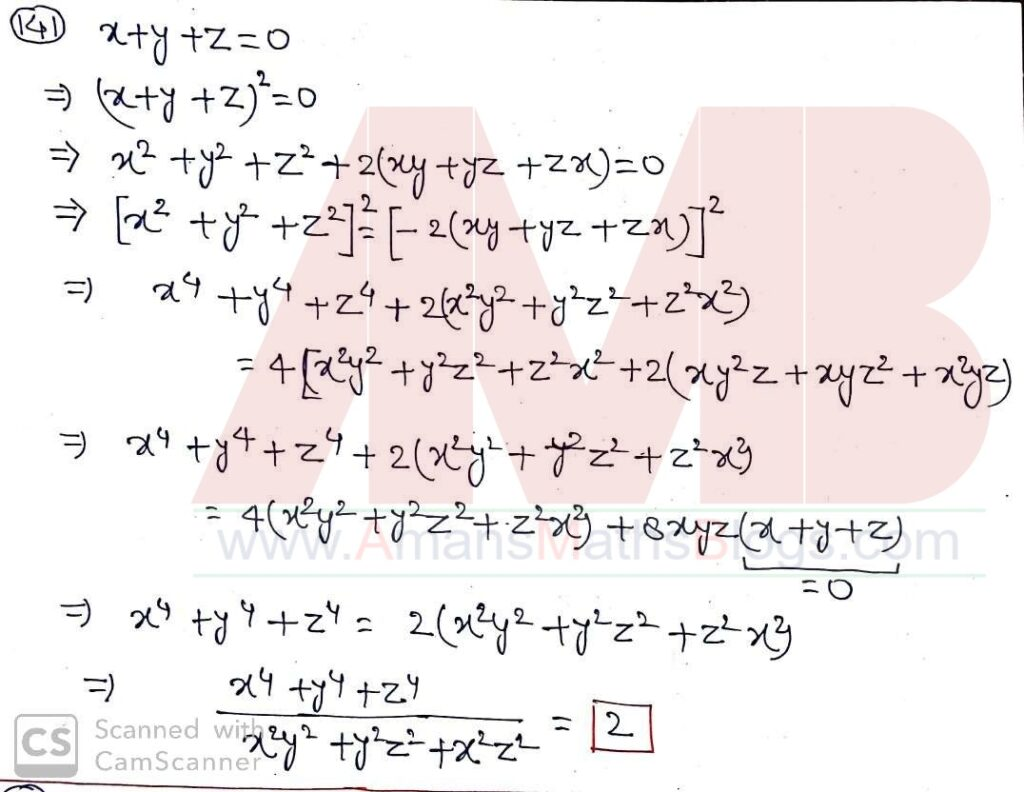JSTSE 2020-21 Question Paper With Answer Keys and Solution: Ques No 142

If xa = y2b = z3c and y2 = zx, then find the value of 1/a + 1/3c is

Options:

1. b/2

2. b

3. 1/b

4. 2/b

Solution: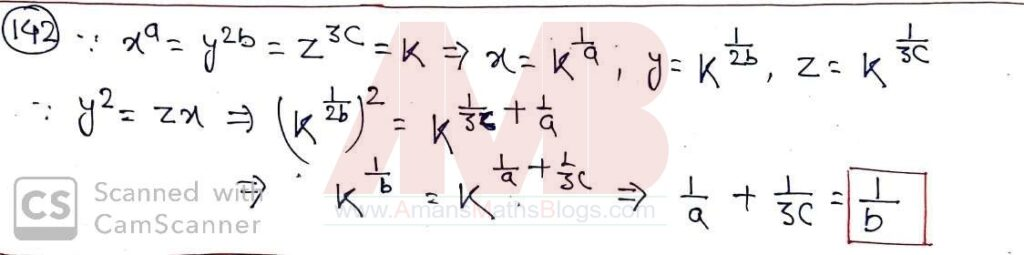MVPP 2020-21 Question Paper With Answer Keys and Solution: Ques No 143

If m = 21/3 + 2-1/3, then find the value of 2m3 – 6m + 1 is

Options:

1. 6

2. 2

3. 3

4. 4

Solution:JSTSE 2020-21 Question Paper With Answer Keys and Solution: Ques No 144

The coefficient of p2 in (p2 + 2p + 3)2 + (p2 – 2p + 3)2 is

Options:

1. 6

2. 10

3. 18

4. 20

Solution: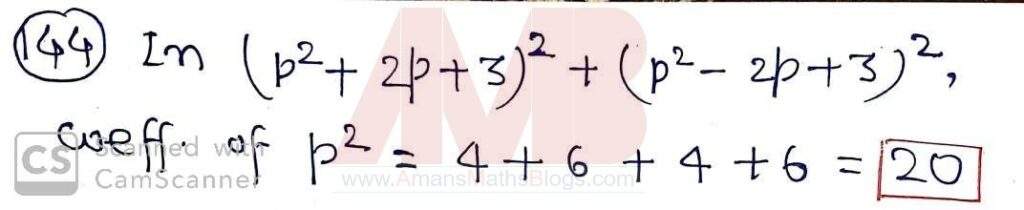MVPP 2020-21 Question Paper With Answer Keys and Solution: Ques No 145

Factorize the following : a3 + 3a2b + 3ab2 + 2b3.

Options:

1. (2a + b)(a2 + ab + b2)

2. (a + 2b)(a2 + ab + b2)

3. (2a + b)(a2 + 3ab + b2)

4. (a + 2b)(a2 + 3ab + b2)

Solution:JSTSE 2020-21 Question Paper With Answer Keys and Solution: Ques No 146

If a = ∛64 and b = ∛65, then find the value of (a + b) – 1/(a2 + ab + b2).

Options:

1. 2

2. 4

3. 8

4. 16

Solution: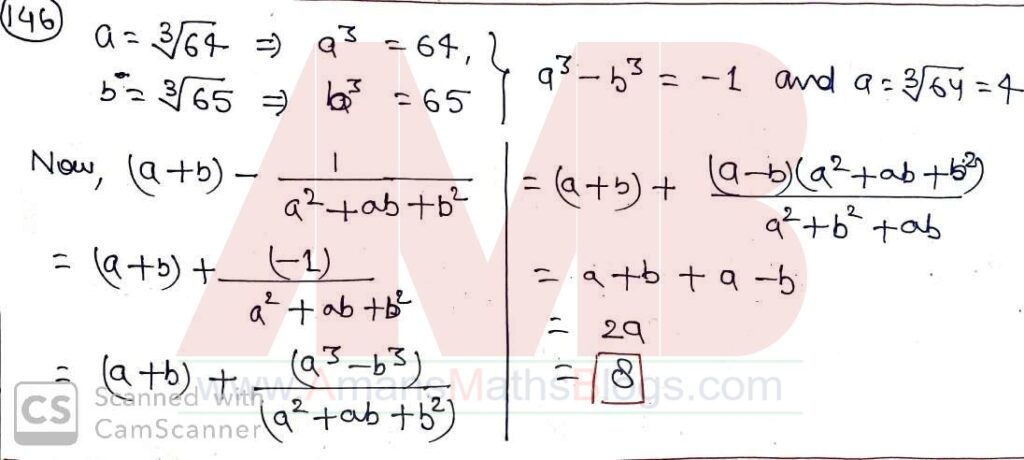MVPP 2020-21 Question Paper With Answer Keys and Solution: Ques No 147

If 9K+2 – 240 = 9K, then the value of (18K)K is

Options:

1. 3

2. 9

3. 3√2

4. 2√3

Solution:JSTSE 2020-21 Question Paper With Answer Keys and Solution: Ques No 148

If z = 3 + 31/3 + 32/3, then find the value of z3 – 9z2 + 18z – 7.

Options:

1. 12

2. 10

3. 5

4. -5

Solution: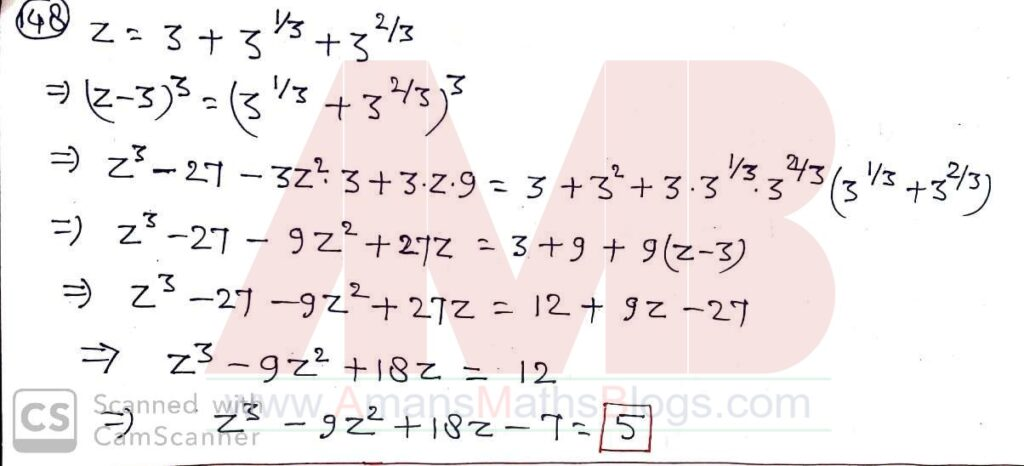MVPP 2020-21 Question Paper With Answer Keys and Solution: Ques No 149

If L : M : N = 2 : 3 : 4 and L2 + M2 + N2 = 11600, then find the value of L + M – N.

Options:

1. 20

2. 60

3. 100

4. 180

Solution: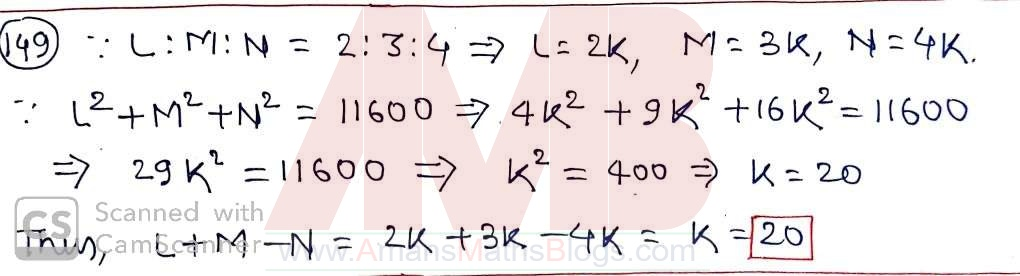JSTSE 2020-21 Question Paper With Answer Keys and Solution: Ques No 150

If p + q + r = 2, pq + qr + rp = -1 and pqr = -2, then find the value of p3 + q3 + r3.

Options:

1. 8

2. -8

3. 16

4. -16

Solution:MVPP 2020-21 Question Paper With Answer Keys and Solution: Ques No 151

If a = √2 – √6, b = √6 – √3 and c = √3 – √2, then find the value of a3 + b3 + c3 – 2abc is

Options:

1. 0

2. √2 + √3 + √6

3. 5√3 – √6 + 3√2

4. 3√2 + √6 – 4√3

Solution:JSTSE 2020-21 Question Paper With Answer Keys and Solution: Ques No 152

Factorize : x2y + xz2 + y2z – x2z – y2x – z2y.

Options:

1. (x + y)(y + z)(z + x)

2. (x – y)(y + z)(z – x)

3. (x – y)(y – z)(z – x)

4. (x – y)(y – z)(x – z)

Solution:MVPP 2020-21 Question Paper With Answer Keys and Solution: Ques No 153

In the given figure, XZ = ZY = XT, then the value of x is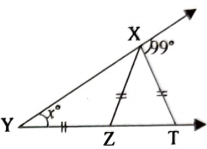Options:

1. 30

2. 33

3. 49

4. 49.5

Solution:JSTSE 2020-21 Question Paper With Answer Keys and Solution: Ques No 154

If a + b = m and 1/a + 1/b = 1/n, then relationship in m, n and a is

Options:

1. m(a – n) = a2

2. m(a + n) = a2

3. a(m – n) = a2

4. a(m + n) = n2

Solution: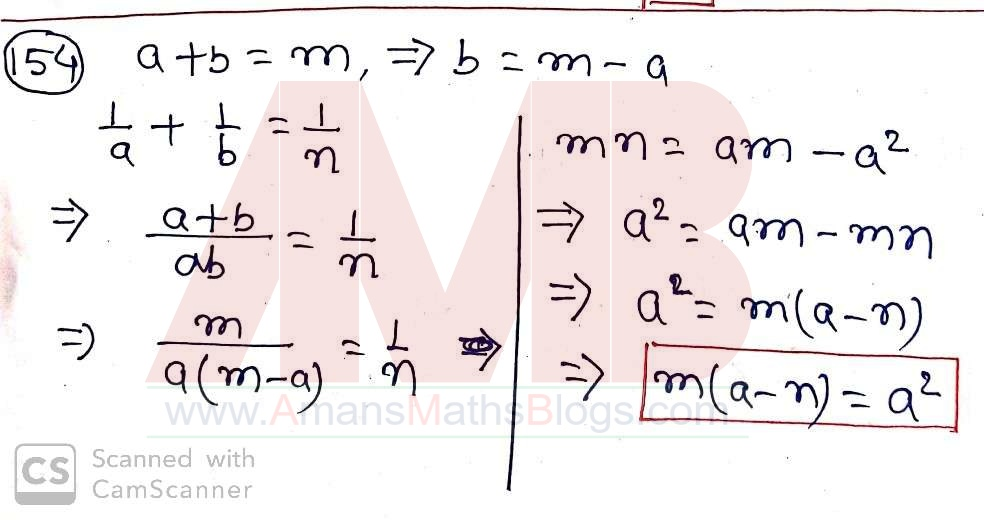MVPP 2020-21 Question Paper With Answer Keys and Solution: Ques No 155

If x = 0.473 (bar over 73), then find the value of x + 1.6 (bar over 6).

Options:

1. 2.140 (bar over 140)

2. 0.2140 (bar over 40)

3. 21.40 (bar over 40)

4. 2.140 (bar over 40)

Solution: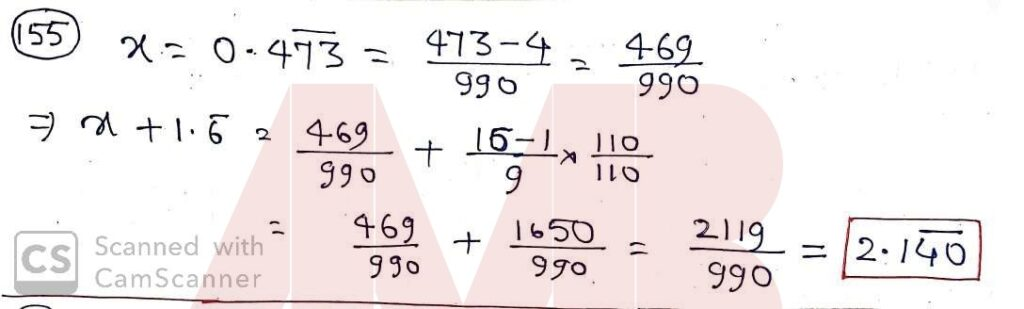JSTSE 2020-21 Question Paper With Answer Keys and Solution: Ques No 156

Area of the quadrilateral REST, in which angle R = 90 degree, RE = 30 cm, ES = 42 cm, ST = 20 cm and TR = 16 cm is

Options:

1. 192 sq. cm

2. 240 sq. cm

3. 336 sq. cm

4. 576 sq. cm

Solution:MVPP 2020-21 Question Paper With Answer Keys and Solution: Ques No 157

A train of length 240 cm, crosses a platform in 20 seconds. If the speed of the train is 72 km/hr., then the length of platform is

Options:

1. 160 m

2. 180 m

3. 240 m

4. 260 m

Solution: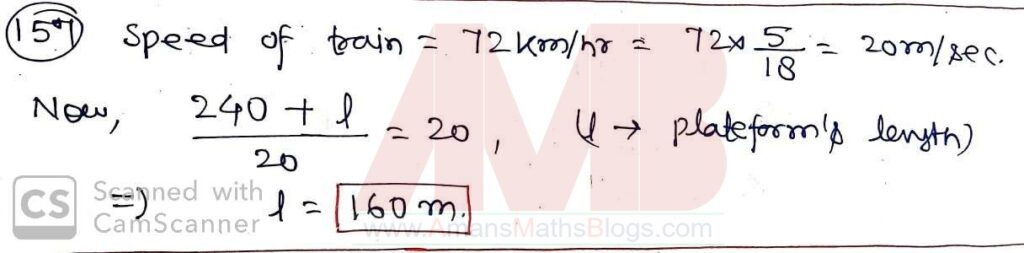JSTSE 2020-21 Question Paper With Answer Keys and Solution: Ques No 158

In given figure, ABC is an equilateral triangle of side 8 cm. Find the area of shaded region.Options:

1. 32 – 16π/3 sq. cm

2. 32 – 8π/3 sq. cm

3. 16√3 – 8π sq. cm

4. 32√3 – 16π sq. cm

Solution: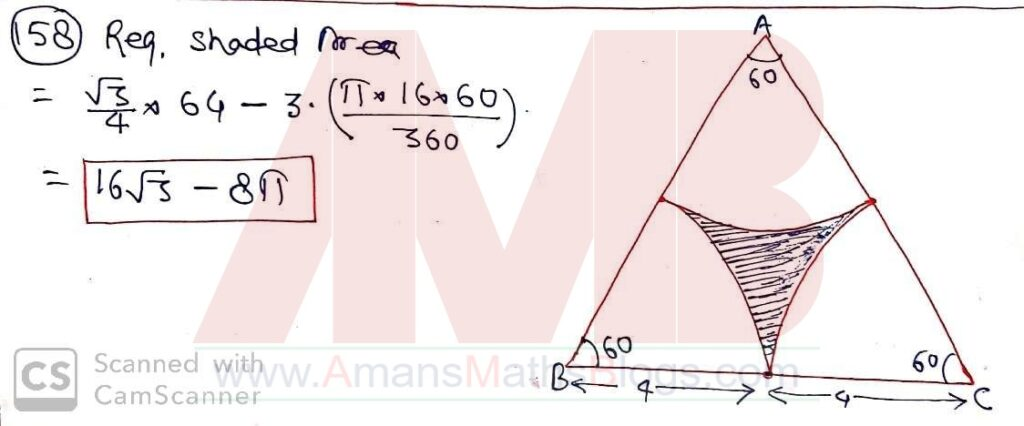MVPP 2020-21 Question Paper With Answer Keys and Solution: Ques No 159

Mean of 9 observation was found to be 35. Later on, it was detected that an observation 80 was missed as 8. The correct mean is

Options:

1. 45

2. 44

3. 43

4. 42

Solution: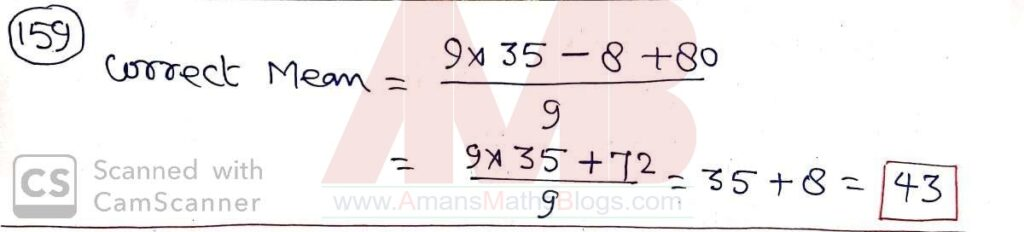JSTSE 2020-21 Question Paper With Answer Keys and Solution: Ques No 160

If (3√2 + 2√3)/(4√2 + 3√3) = p + q√6, then find the value of p and q.

Options:

1. p = 1/5 and q = 6/5

2. p = 6/5 and q = 1/5

3. p = 6/5 and q = -1/5

4. p = -6/5 and q = 1/5